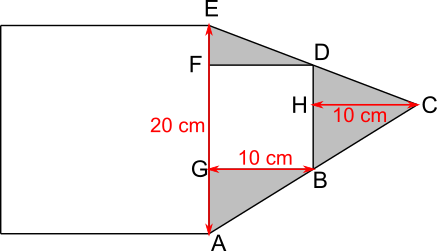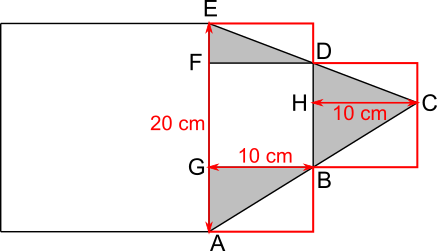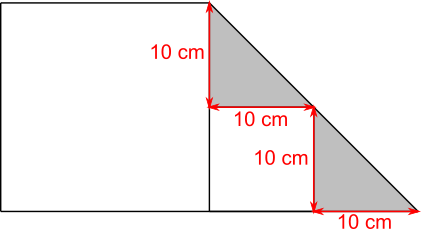#### You may also like### Some(?) of the Parts

A circle touches the lines OA, OB and AB where OA and OB are perpendicular. Show that the diameter of the circle is equal to the perimeter of the triangle### Triangle Midpoints

You are only given the three midpoints of the sides of a triangle. How can you construct the original triangle?### Fermat's Poser

Find the point whose sum of distances from the vertices (corners) of a given triangle is a minimum.

# Block Challenge

##### Age 14 to 16 Short Challenge Level:

Using the large triangleThe shaded area is equal to the area of triangle ACE subtract the area of the smaller square, which is 10$\times$10 = 100 cm$^2.$

The 'base' of triangle ACE is AE = 20 cm, the same as the side of the larger square.

AC is a straight line, so triangles ABG and BCH are similar. Triangles FDE and HCD are also similar.

AG + GF + FE = AE = 20 cm, so since GF is 10 cm, AG and FE add up to 10 cm. So, since BH and HD also add up to 10 cm, triangles ABG and BCH are congruent. GB is 10 cm because it is the side of the smaller square, so HC is also 10 cm.

This means that the 'height' of triangle ACE is 20 cm, so the area is $\frac12$20$\times$20 = 200 cm$^2.$

So the shaded area is 200 cm$^2-$100 cm$^2$ = 100 cm$^2.$

Using small trianglesAE = 20 cm because it is the side length of the larger square.

AC is a straight line, so triangles ABG and BCH are similar. Triangles FDE and HCD are also similar.

AG + GF + FE = AE = 20 cm, so since GF is 10 cm, AG and FE add up to 10 cm. So, since BH and HD also add up to 10 cm, triangles ABG and BCH are congruent. FG is 10 cm because it is the side of the smaller square, so HC is also 10 cm.

This means that the rectangle enclosing triangle DBC is a square of side length 10 cm, and, since AG + FE = 10 cm, the rectangles enclosing triangles ABG and FDE fit together to make a square of side length 10 cm.

Since the triangles occupy half of the rectangles, the shaded area is equal to half of the area of two squares of side length 10 cm. That is the same as the area of one square of side length 10 cm, so 10 cm$\times$10 cm = 100 cm$^2.$

Using exam technique
This method only works if we assume that the question-setter knew the question was answerable

Because the lengths above and below the smaller square are not given, we can assume that changing them does not affect the shaded area.

This means that the shaded area would still be the same if the base of the smaller square was level with the base of the larger square, as in the diagram below.The base of the upper triangle is 10 cm because it is the side length of the smaller square. The height is also 10 cm as it is the difference between the side lengths of the two squares, 20 cm$-$10 cm. Since the height of the lower triangle is also 10 cm, the base must be 10 cm too, because the two triangles are similar.

The area of each triangle is $\frac{1}{2}\times$10$\times$10 = 50 cm$^2,$ so the total shaded area is 100 cm$^2.$
You can find more short problems, arranged by curriculum topic, in our short problems collection.